Next: Implementation Up: Inflation Previous: Inflation   Contents

Mathematical background

Let a system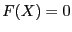,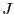the Jacobian matrix of this system and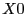a solution of the system. The purpose of the inflation method is to build a box that will contain only this solution. Let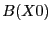be a ball centered at: if for any point in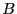is not singular, then the ball contains only one solution of the system.

The problem now is to determine a ball such for any point in the ball the Jacobian is regular. Let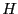be the matrix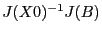whose components are intervals. Let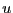be the diagonal element of H having the lowest absolute value, let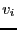be the maximum of the absolute value of the sum of the elements at row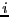of, discarding the diagonal element of the row and let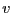be the maximum of the's. If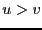, then the matrix is denoted diagonally dominant and all the matrices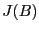are regular .

Let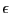be a small constant: we will build incrementally the ballby using an iterative scheme defined as: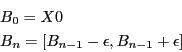that will be repeated until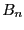is no more diagonally dominant.Note that in some cases (see for example section 2.16) it is possible to calculate directly the largest possibleso that all the matrices in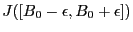are regular without relying on the iterative scheme.Next: Implementation Up: Inflation Previous: Inflation   Contents
Jean-Pierre Merlet 2012-12-20